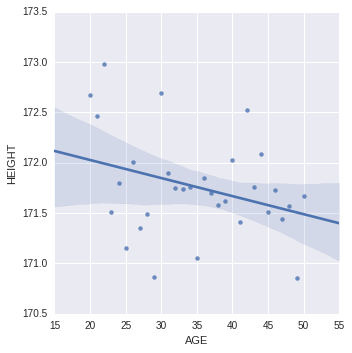# samucoder

Samuel M.H. 's technological blog

Notebook

# Pearson Correlation¶

Author: Samuel M.H. <samuel.mh@gmail.com> Date: 31-01-2016

## Instructions

Generate a correlation coefficient.

Note 1: Two 3+ level categorical variables can be used to generate a correlation coefficient if the the categories are ordered and the average (i.e. mean) can be interpreted. The scatter plot on the other hand will not be useful. In general the scatterplot is not useful for discrete variables (i.e. those that take on a limited number of values).

Note 2: When we square r, it tells us what proportion of the variability in one variable is described by variation in the second variable (a.k.a. RSquared or Coefficient of Determination).

## What to submit:

Following completion of the steps described above, create a blog entry where you submit syntax used to generate a correlation coefficient (copied and pasted from your program) along with corresponding output and a few sentences of interpretation.

## Dataset

In :
%matplotlib inline

import pandas
import matplotlib.pyplot as plt
import scipy
import seaborn as sns


## Test of correlation

I am testing if there is a linear relationship between the age (years) and the average height (cm) in the population of white people between 20 and 50 years old.

### Ingesting and curating the data

In :
#Load
#Select
data = data[(data['AGE']>=20) & (data['AGE']<=50) & (data['ETHRACE2A']==1)]
data = data.dropna()
print(data.shape)

(13190, 4)



So far, we have 13.190 samples.

In :
#Create the dataframe with the required features
df1 = pandas.DataFrame()
df1['AGE'] = data['AGE']
df1['HEIGHT'] = (data['S1Q24FT']*12 + data['S1Q24IN'] )* 2.54


### Detecting outliers

In :
df1.describe()

Out:
AGE HEIGHT
count 13190.000000 13190.000000
mean 36.038817 221.337603
std 8.650123 388.738953
min 20.000000 132.080000
25% 29.000000 165.100000
50% 37.000000 172.720000
75% 43.000000 180.340000
max 50.000000 3268.980000

Obviously there are measurement errors (a person cannot be 32m height), lets say there is no person taller than 250 cm.

In :
df1 = df1[ df1['HEIGHT']<=250 ]
print('Corrected Max height: {0}cm'.format(df1['HEIGHT'].max()))

Corrected Max height: 213.36cm



### Aggregating by year

Now I compute the average height per year group.

In :
averages = df1.groupby('AGE', as_index=False).mean()
sns.lmplot(x='AGE', y='HEIGHT', data=averages)

Out:
<seaborn.axisgrid.FacetGrid at 0x7f986b5b2990>A negative linear correlation can be seen in the plot, but... how significant is it?

### Linear Correlation

I calculate the pearson correlation between the age and the height.

In :
r,p = scipy.stats.pearsonr(averages['AGE'],averages['HEIGHT'])
print('Correlation coefficient (r): {0}'.format(r))
print('p-value: {0}'.format(p))

Correlation coefficient (r): -0.32178210607
p-value: 0.0775178076391



There is a negative weak correlation and the p-value is bigger than 0.05 so it is safe to say that there is NO statistically significant linear correlation between the age and the height. The weak correlation means that it could happen by chance.